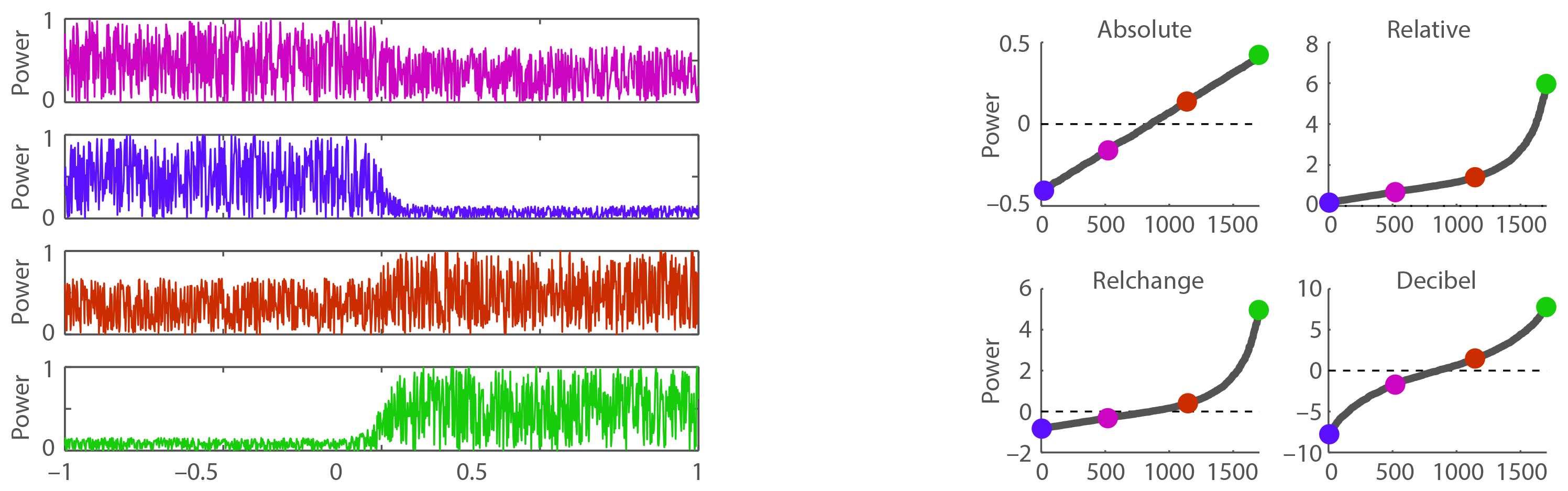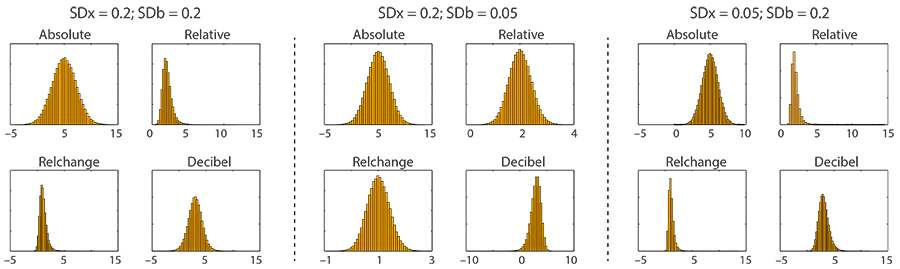Baseline correction

Electroencephalography (EEG) and magnetoencephalography (MEG) signals (among others) might drift over time and different EEG/MEG channels might exhibt such drifts and/or DC (direct current) offsets differently. These drifts and offsets can be due to sweating and skin conductances or other noise sources. In order to investigate the neural response to an event one would thus like to remove these "uninteresting" signals.

The term baseline correction in electroencephalography (EEG) and magnetoencephalography (MEG) refers to the procedure of relativizing the brain signal of interest with respect to a control (baseline) signal (commonly shortly before a stimulus event). It is a very common procedure for the analysis of time-domain data (e.g., event-related potentials; ERPs) or time-frequency data (i.e., power obtained from spectral decomposition using, for example, wavelet convolution or Fourier transformation). However, in particular for time-frequency data, there is no consensus on which baseline correction should be used. For time-domain signals, the 'absolute' baseline is commonly used.

Baseline Correction: Types

Common types of baseline corrections are (in Matlab syntax, X being the signal and B the baseline):

 absolute baseline range: [-inf inf] X - B relative baseline range: [0 inf] X / B relative change (relchange) baseline range: [-1 inf] (X-B) / B decibel baseline (this baseline is defined only for power) range: [-inf inf] 10*log10(X / B)

For an additional approach see Grandchamp & Delorme (2011). For time-domain signals, the absolute baseline is the most common correction and should be the default. For time-frequency data, there is not yet a consensus in the literature. Based on the simulations below, the decibel baseline appears to have good properties. Please also see Urbach & Kutas (2006) who have commented on the potential problems using baseline corrections.

In some cases one might be able to replace the time-domain baseline correction by using a high-pass filter with a strong DC (direct current) suppression. Papers making use of this approach are, for example, Maess et al. (2006) or Herrmann et al. (2014).

Baseline Correction: Distributions

Baseline corrections might introduce skewed data distributions. The "relative" baseline and the "relchange" baseline appear to be particularly prone to introduce skewed (non-Gaussian) data distributions. This might affect data plotting and statistics: linear plotting and parametric statistics might be inappropriate. For the following simulations, the power in the baseline time window (-1 to 0 sec.) relative to the power in the time window of interest (0 to 1 sec.) is linearly changed for 1702 power time courses. For half of the time courses, the mean baseline power is larger than the mean power for the time window of interest. For the other half, the mean baseline power is smaller. Four example time courses (out of 1702) are shown on the left.On the right, the mean baseline-corrected power is plotted for the 1702 power time courses (separately for each baseline). The mean baseline-corrected power of the four time courses (displayed on the left) are color-coded. The blue and green dots are of particular interested (a large power decrease relative to the baseline [blue] vs. a large power increase relative to the baseline [green]). Although the change for the blue and green time courses are of similar magnitude, the mean baseline-corrected power for the relative and relchange baselines lead to drastic magnitude differences. This is because if the mean baseline power is large relative to the power in the time window of interest, the baseline-corrected power is squeezed into a small range (0 to 1 for relative; -1 to 0 for relchange). This simple simulation shows that depending on the type of baseline correction, the distribution of baseline-corrected power values can be skewed (for further simulations on this see next the section). In this regard, the 'absolute' and 'decibel' baseline corrections have better properties than the 'relative' and 'relchange' baseline corrections.

Baseline Correction: Distributions might depend on variance

For additional simulations on distributional changes introduced by baseline corrections, you might be interested in this document which is based on this simulation script. Some of the simulation results are reproduced here:The plots above show distributions (histograms) of power values for different baseline correction types. The histograms are based on a signal distribution (N=100000; Gaussian noise, with a mean of 10) and a baseline distribution (N=100000; Gaussian noise, with a mean of 5). Standard deviations (relative to the mean; for details see the matlab script) for the distributions were varied. The plots above reflect the changes in the distribution depending on the standard deviantions. In particular the power values for relative and relchange baselines can have very skewed distributions after the correction.

Baseline Correction: Single Trials vs. Average

Depending on the research question, single trials or the average across trials might be analyzed. Importantly, baseline correction will produce different results depending on the stage at which the correction is applied (except for the "absolute" baseline). Baseline correction can be applied on single trials before trials are averaged, or trials are averaged first and baseline correction is applied on the averaged signal.

The comparison between the "baseline first" (baseline correction on single trials and then average across trials) versus "baseline second" (average across trials followed by baseline correction) approaches is shown in the following example (Matlab syntax). In the example, X contains two trials (rows) and four time points (columns). The average across the first two of the time points are considering the baseline. Xm is the average across trials, and Bm the baseline for the trial average.

Example code:
X = [1 2 3 4; 4 5 6 7] % two trials with 4 time samples; rows refer to trials
B = mean(X(:,[1 2]),2) % the mean of the first 2 time samples = baseline
Xm = mean(X,1) % across-trial average
Bm = mean(B,1) % baseline for across-trial average
B = repmat(B,1,size(X,2)) % in order for matlab to work, B needs to have the same dimensions as X

% 'absolute' baseline
mean(X – B) == Xm-Bm % same

% 'relative' baseline
mean(X ./ B) ~= Xm./Bm % different

% 'relchange' baseline
mean((X-B) ./ B) ~= (Xm-Bm)./Bm % different

% 'decibel' baseline (this baseline is defined only for power)
mean(10*log10(X ./ B)) ~= 10*log10(Xm./Bm) % different